# Program to check Unique Number In Java

In this tutorial you will learn about the Program to check Unique Number In Java and its application with practical example.

In this tutorial, we will learn to create a Program to check Unique Number  in Java using Java programming.

## Prerequisites

Before starting with this tutorial we assume that you are best aware of the following Java programming topics:

• Java Operators.
• Basic Input and Output function in Java.
• Class and Object in Java.
• Basic Java programming.
• If-else statements in Java.
• For loop in Java.

### What is Unique Number.

The number is called a unique if there is no repeated digits in the given number.

For Example:=> 20, 56, 98 are Unique Number
But => “22,99,121,100” are not unique because they contain repeated digits in them.

## Program to check Unique Number In Java

In this program we would find the given number is a Unique number or not .first of all we would take a value from user and find the Unique number.let have a look at the code.

## Output

Unique Number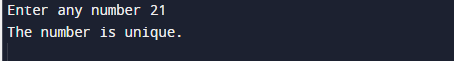Not a Unique Number.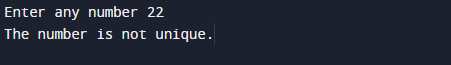In the above program, we have first declared and initialized a set variables required in the program.

• number= it will hold entered number.
• num1,num2=it will hold temporary number.
• count=   it will hold repetition number,
• rem,rem1- it will hold last digit of a numbers.

After that we take a number from user and find given number is a Unique number or not.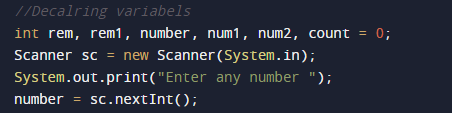Steps to check given number is unique or not:

1. take a value  from the user in number variable.
2. Break digit and find the last digit o the number.
3. Check each digit in number with the last digit.
4. If the digit repeated more than one time, the number is not unique.
5. Else, remove the last digit of the number.
6. Repeat steps until the number becomes zero.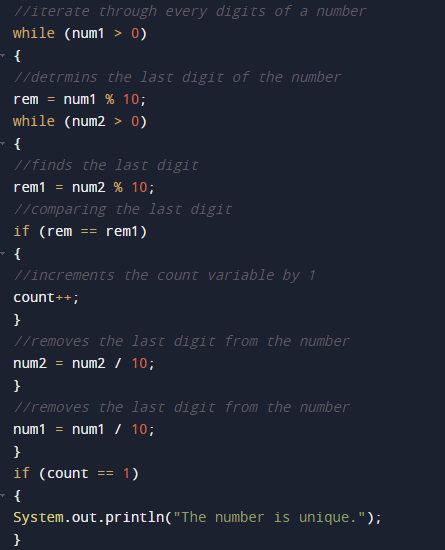The number is called a unique if there is no repeated digits in the given number.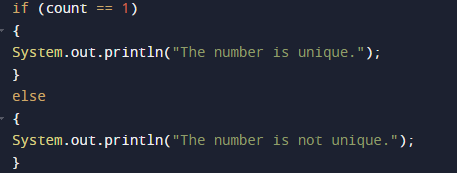else it is not a unique number.

In this tutorial we have learn about the Program to check Unique Number In Java and its application with practical example. I hope you will like this tutorial.